# NEET Physics 2021

NEET Physics syllabus from class 11 and 12 has 6 prime units – Modern Physics,  Mechanics, Thermodynamics, Electrodynamics,  SHM-Waves and Optics. 45 out of 180 questions asked in NEET are from the NEET Physics section. In comparison to Biology and Chemistry, students often have a tough time dealing with Physics primarily because of the typology of questions appearing in this section. It consists of computations of numericals, theoretical and application types of questions and more.

NEET Physics necessitates round-the-clock practice coupled with an in-depth comprehension of concepts and associated formulae. In order to score well in NEET Physics, firstly learn and understand all important topics, including formulae as specified in the syllabus. This would be the first step to crack challenging numericals effortlessly. Physics numericals are deemed no less than a nightmare by students because of which they tend to overlook it and do not practise enough. As a result, they focus on strengthening other sections of NEET. This has been perceived as the major reason why students fail to score as much in NEET Physics, affecting their final NEET score.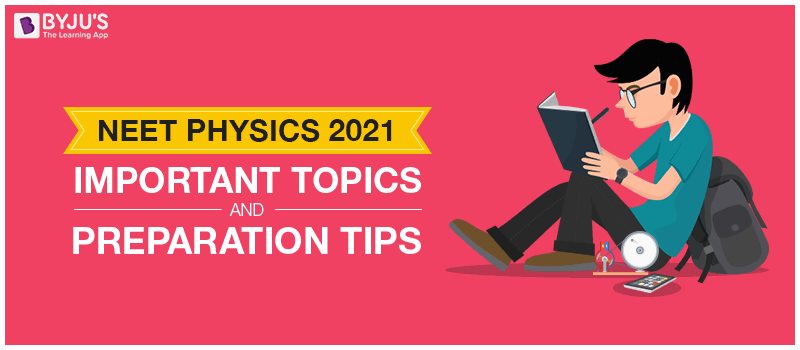One of the most common practices amongst NEET aspirants that has been observed is the rote learning technique, where one memorizes. While the approach has gained momentum in Chemistry and Biology section, helping students, the same cannot be applied when it comes to NEET Physics section. To get a thorough insight into your learnings, it is suggested to take up test series provided topic-wise for every topic and hence, correlating with its application practically.

Also Read: Physics MCQs for NEET#### Elastic property of Matter#### Angular Momentum of Rigid Body#### Parallel Axis Theorem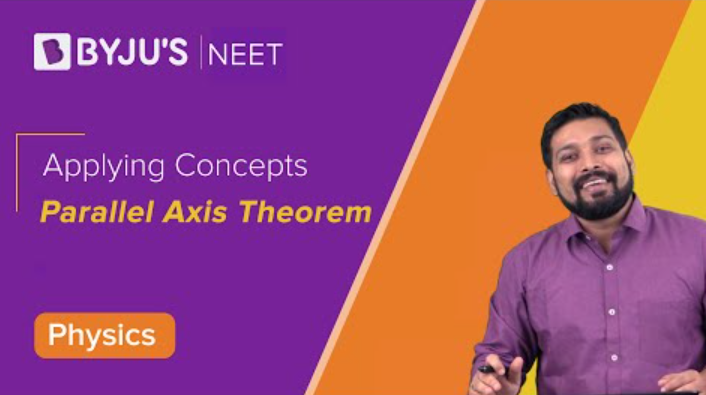#### Perpendicular Axis Theorem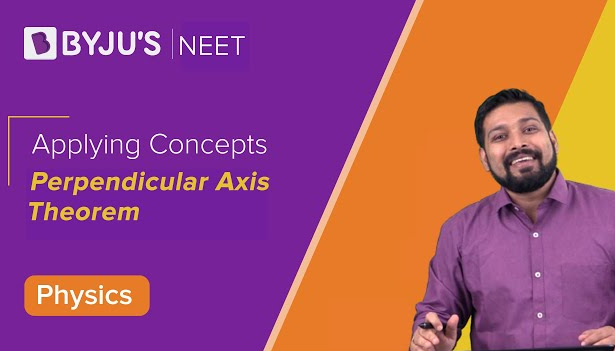#### Application of Circular Motion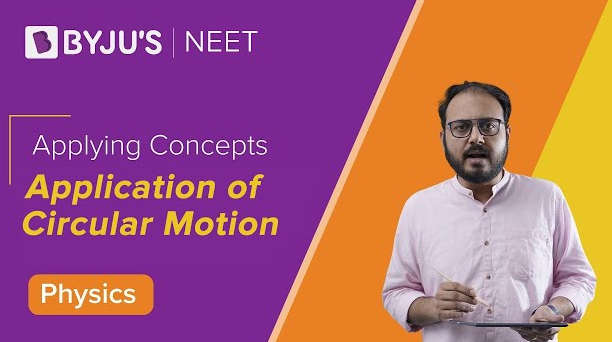#### Non Uniform Circular Motion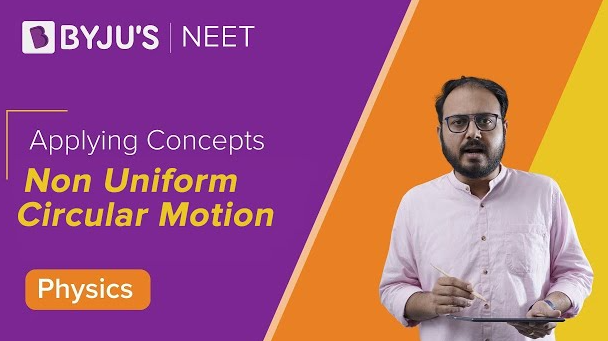## NEET Physics 2020 Analysis

Given below is topic-wise weightage as seen in NEET 2020 Physics section. Take a look:

 Name of Unit Weightage (approximate) Physical World and Measurement 2% Kinematics 7% Laws of Motion 7% Work, Energy and Power 4% System of Particles and Rotational Motion 4.50% Gravitation 4.50% Properties of Bulk Matter 9% Oscillations and Waves 7% Thermodynamics 4.50% Behaviour of Perfect Gas and Kinetic Theory 2% Electrostatics 4% Current Electricity 9% Magnetic Effects of Current and Magnetism 7% Electromagnetic Induction and Alternating Currents 4% Electromagnetic Waves 2% Optics 4.50% Dual Nature of Radiation and Matter 4% Atoms and Nuclei 7% Electronic Devices 7%

Compared to previous years, NEET Physics 2020 was relatively easier. Close to 33% of the Physics section was based on NCERT. Out of all the units, Mechanics carried the highest weightage. 12 questions were asked from the unit.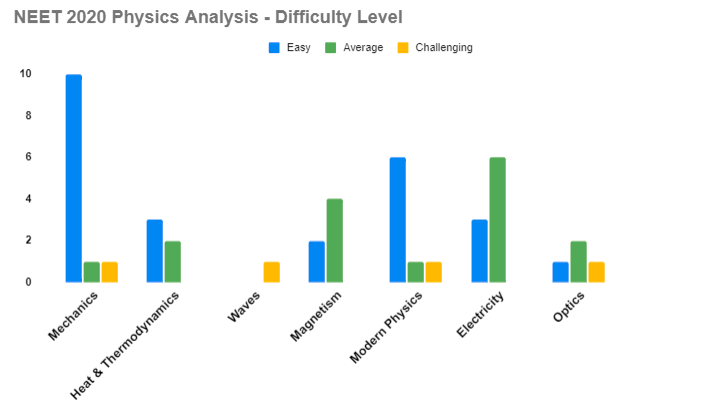Graph indicating Chapter name vs. the number of questions asked, segregated as per difficulty level (NEET 2020)

## NEET Physics 2019 Analysis

NEET 2019 Physics section was deemed average in terms of complexity and length. Several questions were formula based. To crack the section with good marks, a strong and solid hold on concepts with its prompt application was inevitable. Aspirants found most of the questions appearing from NCERT syllabus, precisely close to 60%. The Physics section in particular was found to be comparatively easier. NEET 2019 was considered to be easier than the previous years. Most of the questions were asked from the Mechanics and Modern Physics section

Equal distribution of questions from both classes 11 and 12 was also taken note of. The topics that dominated NEET Physics section were Modern Physics and Mechanics, almost 50% of the section. Easier questions appeared from units such as Current Electricity and Magnetic Effect of Current. Also, since the paper for NEET 2019 was easier, there were high chances for the NEET cut-off to have increased.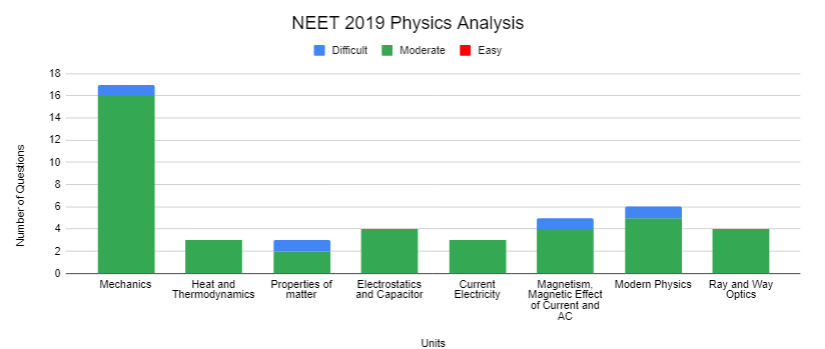Given below is the NEET Physics 2019 Analysis chapter-wise:

 Name Of The Unit Number of Questions Weightage Marks Current Electricity 3 6.66% 12 Electrostatics and Capacitor 4 8.88% 16 Heat and Thermodynamics 3 6.66% 12 Magnetism, Magnetic Effect of Current and AC 5 11.11% 20 Mechanics 17 37.82% 68 Modern Physics 6 13.33 24 Properties of matter 3 6.66% 12 Ray and Way Optics 4 8.88% 16 Total 45 100 180

Also Check: NEET 2021 Syllabus

## NEET Physics Preparation Tips:

Mechanics:

Topping the list of the top-scoring chapters for Physics, Mechanics, as seen above, is clearly one of the most important units. 17 out of 45 questions appeared from the unit alone. It, in turn, comprises 9 chapters – Class 11 from Chapter Units and Dimensions to Chapter Oscillations and Waves.

Find below the list of chapters categorized under Mechanics along with the corresponding weightage (previous trends):

 Chapter Name Weightage Gravity 10.70% Kinematics 13% Laws of Motion 7.10% Mechanics of Solids and Fluids 10.10% Oscillations 2.40% System of Particle and Rotational Motion 23.10% Units and Dimensions 7.77% Waves 18.30% Work, Energy and Power 7.70%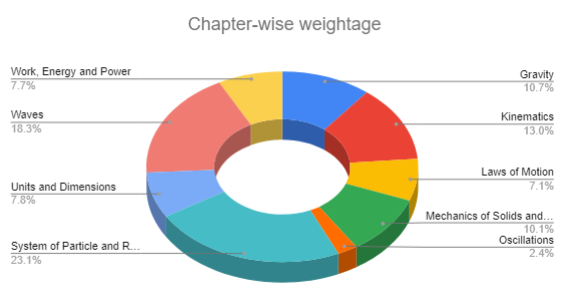A few important concepts covered in Mechanics are:

 Dimensional and error analysis Acceleration due to gravity Conservation of momentum & energy and collisions Equations of motion Kepler’s laws Mechanics of common forces Moment of inertia and dynamics of rotational motion Pascal’s law, Bernoulli’s principle, viscosity and Reynolds’ number Potential energy of a spring Projectile and circular motion Relative motion Satellites Simple Harmonic motion Standing waves & Beats, Doppler effect Transverse and longitudinal waves: superposition and reflection

Heat and Thermodynamics:

Every year, around 3 questions appear from this unit, carrying a weightage of 6.6%.

The table below depicts the chapters under this unit:

 Chapter Name Weightage Thermodynamics 63.2% Thermal Properties 33.3% Kinetic theory of gases 3.5%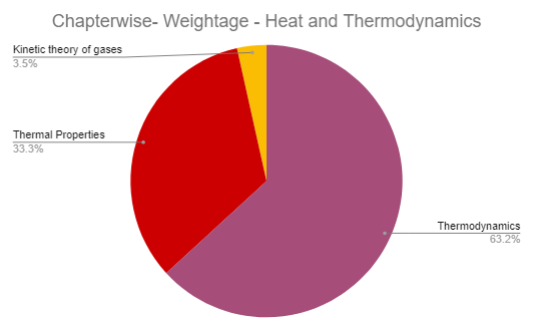A few important concepts covered in Heat and Thermodynamics are:

 First Law of Thermodynamics and Heat Capacity Heat engines, refrigerators and heat pumps Heat transfer Law of equipartition of energy, specific heat capacity and mean free path Newton’s Law of Cooling Reversible and irreversible processes and Carnot engine Second law of thermodynamics Specific Heat Capacity and Calorimetry Thermodynamic state variables, equation of state and thermodynamic processes Transfer of Thermal Expansion

Electrostatics and Magnetism

This unit carries a weightage of 16.2% and the chapters are interrelated. Under this unit, the chapter Electrostatic potential and capacitance weighs nearly 35.6%, followed by Moving charges and Mechanism weighing around 31.5%. The unit majorly covers important numericals that appear in the examination. Listed below are some topics which should not be missed at all:

• Electrostatic potential and work done
• Motion in electric and magnetic fields
• Potential energy in an external field
• Cyclotrons and Torque on Current loop
• Magnetic dipole

Also Explore:

Here is a list of chapters and the corresponding weightage of chapters under this unit:

 Chapter Name Weightage Electric charges and fields 23.30% Electric potential and capacitance 35.60% Electromagnetic waves and communication systems 6.80% Magnetism and matter 2.70% Moving charges and magnetism 31.50%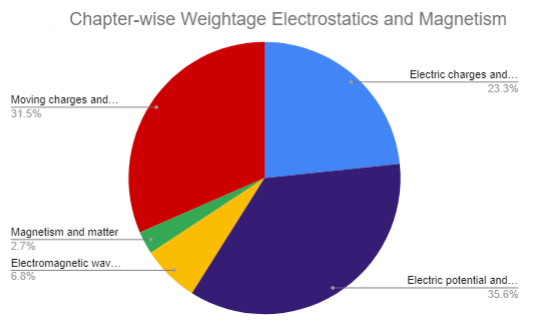Some significant topics are:

 Ampere’s Circuital law Bandwidth and modulation of signals in communication systems Bar magnet and magnetic field lines Biot-Savart Law Capacitance and Capacitors Continuous charge distribution and Gauss’s Law Electric charge and Coulomb’s Law Electric field, field lines, flux Electromagnetic waves and spectrum Electrostatic potential and work done Electrostatics of conductors and Dielectrics Force between two parallel currents Motion in electric and magnetic fields and Cyclotrons Potential energy in an external field The earth’s magnetism Torque on current loop and magnetic dipole

### Kinematics:

Kinematics is one of the most interesting and crucial units of NEET Physics. The problems based on the evaluation of the maximum height, range, velocity and time of flight (2 D motion) are important from the examination point of view. Maintain proper notes of all the theorems, formulas, and derivations to assist your last-minute preparations. Strengthen your concepts on acceleration, displacement, average, and relative velocity to score well from this unit.

The students can follow NCERT books for NEET to strengthen their theoretical knowledge. For numerical problems, the students can refer to solved problems in Physics by Schaum. Concepts of Physics by H C Verma (Vol 1 & Vol 2) is helpful for both theoretical and numerical physics.

Stay tuned with BYJU’S to know more about NEET  eligibility, exam pattern, application form, preparation tips, previous years’ question papers, sample papers, NEET Physics study materials and more.

Note: The inferences discussed in the aforementioned tables are an average, cumulative from previous years’ question papers. Data in these tables is an average, (i.e., weightage, number of questions) they are approximate values and subject to vary.

## Frequently Asked Questions on NEET Physics

### How To Study Physics For NEET?

1. Be well-versed with NEET syllabus and exam format
2. Master NCERT, gain conceptual clarity
3. Prepare notes, make a list of formulae and derivations
4. Solve as many previous years’ question papers
5. Practise from exemplars and other relevant reference books, solve as many questions as possible
6. Take up a mock test every day
7. Focus on strengthening weak areas
8. Improve speed and accuracy levels by practising as much as possible

### Which Book Is Best For NEET Physics?

NCERT must be mastered. Apart from that one can refer to Concepts of Physics by H.C Verma, D. Mukherjee for MCQs, DC Pandey, BM Sharma (JEE Advanced version), NCERT Exemplar, Irodov and Krotov (for specific topics), coaching modules, previous years’ papers.

### How To Score 160 In Physics NEET?

NEET Physics section comprises 45 questions, each carrying 4 marks. Hence, to score 160 and above, one must get at least 40 questions right. NEET physics is the most challenging section, to crack it, one must practise as many questions. Get well-versed with the format by referring to previous years’ papers to get an idea of the difficulty level.
1. Gain conceptual clarity
2. Prepare a list of formula and derivations
3. Pick the right resources. Refer to reference books for NEET, some of them are – Concepts of Physics by H.C Verma, D. Mukherjee for MCQs, DC Pandey, BM Sharma (JEE Advanced version), NCERT Exemplar, Irodov and Krotov (for specific topics), NCERT at your fingertips
4. Practising is key in Physics. Solve previous years’ papers
5. Take up mock tests
6. Improve your levels of speed and accuracy by practising at least 150-200 questions every day

### Which Chapters Are Important In Physics For NEET?

Important chapters can be decided based on two factors – difficulty level and frequency of questions from the previous years’ paper analysis. Some important concepts are:

Mechanics, Current electricity and electromagnetic induction, Heat & Thermodynamics, Electrostatistics & Magnetism, Ray Optics, Modern Physics, Rotational motion, SHM & Waves, Electronics

### Is NCERT Fingertips Physics Enough For NEET?

NCERT Physics at your fingertips is a good book for beginners, helps get a solid foundation. Helps enhance skills and easy memorization of formulae for questions based on formulae. But if you are looking at scoring 600+, you need to refer to other books as well, such as HC Verma, SL Arora, NEET Guide, MTG Books, MTG AIIMS Assertion, and Reasons.

### Is NCERT Physics Enough For NEET?

As per students and even experts, NEET Physics is the most challenging section and demands conceptual clarity. NCERT Physics covers concepts and some numericals. Hence, NCERT books alone will not suffice as one needs to solve and practise more questions to secure a good rank.

1. very useful explanation.

2. Love it . Thankyou so much

3. Tayyaba khan

Thanks for this explanation.

4. Namita

Very useful information. Thank you soooo much

5. Very clear cut details. Thank u so much 👍

6. samiksha

Very well explained,,, hats off to u

7. Riza pathan

Thank you so much

8. Very useful to analyse the important topics, that too with the important concepts covered
thank you

9. Atharva Joshi

The clarity of explanation and the list of resources enlisted are fabulous and helpful.

10. Arsalah Ara

Great explanation for NEET physics

11. Very useful 👍

12. thank you so much

13. Very deeply analysis is given, I like it 😊😊😊😊😊😊😊😊